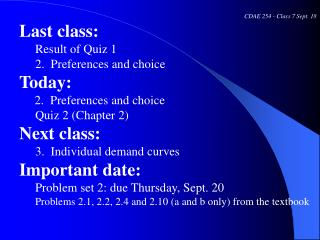DownloadDownload PresentationCDAE 254 - Class 7 Sept. 18 Last class: Result of Quiz 1 2. Preferences and choice Today:

# CDAE 254 - Class 7 Sept. 18 Last class: Result of Quiz 1 2. Preferences and choice Today:

Download Presentation## CDAE 254 - Class 7 Sept. 18 Last class: Result of Quiz 1 2. Preferences and choice Today:

- - - - - - - - - - - - - - - - - - - - - - - - - - - E N D - - - - - - - - - - - - - - - - - - - - - - - - - - -
##### Presentation Transcript

1. CDAE 254 - Class 7 Sept. 18 • Last class: • Result of Quiz 1 • 2. Preferences and choice • Today: • 2. Preferences and choice • Quiz 2 (Chapter 2) • Next class: • 3. Individual demand curves • Important date: • Problem set 2: due Thursday, Sept. 20 • Problems 2.1, 2.2, 2.4 and 2.10 (a and b only) from the textbook

2. 2. Utility and choice 2.1. Basic concepts 2.2. Assumptions about rational choice 2.3. Utility 2.4. Indifference curve and substitution 2.5. Marginal utility and MRS 2.6. Special utility functions 2.7. Budget constraints 2.8. Utility maximization 2.9. Applications

3. 2.8. Utility maximization 2.8.1. A graphical analysis -- Budget constraint -- Indifference curves -- Utility maximization problem -- Solution: Slope of the budget constraint is equal to the slope of the indifference curve: What will happen if the two are not equal? For example, MRS = 1 and Px/Py = 1/2 = 0.5 MRS = 0.3 and Px/Py = 1/2 = 0.5

4. Utility maximization optimum bundle, e, where highest indifference curve touches the budget line

5. Class exercise 3(Thursday, Sept. 13) At the point where the budget line, 2 X + 3 Y = 18, crosses an indifference curve, the MRS = 2. How should the consumer change her consumption of X and Y to maximize her utility (i.e., increase X and decrease Y or decrease X and increase Y)?

6. 2.8. Utility maximization 2.8.2. Graphical analysis – corner solutions --What is a corner solution? -- Possible reasons for corner solutions (a) Due to constraints (e.g., relative prices) (b) Due to preference (e.g., religion reason) -- Business and policy applications

7. 2.8. Utility maximization 2.8.3. A mathematical analysis Maximize U = U (X, Y) subject to Px X + PyY < I

8. 2.9. Applications 2.9.1. Food stamp program in the U.S. (1) How does it work? (2) Economic analysis: income vs. food subsidies (3) Justifications for food subsidies

9. Food Stamps Versus Cash Subsidy All other goods per month Budget line with cash Y + 100 f C e 3 Y I d 2 I 1 I B Budget line with food stamps A Original budget line 0 100 Y Y + 100 Food per month

10. 2.9. Applications 2.9.2. Special cases of utility maximization -- X and Y are perfect substitutes -- X and Y are perfect complements -- X is a useless good -- X is an economic bad -- Consumption quota -- China’s double price system -- Electricity pricing -- A a minimum charge for taxi service -- A company requires its workers to purchase its product

11. Take-home exercise (Tuesday, Sept. 18) While city B faces a likely shortage of electricity in the summer and a surplus of electricity in the winter, the following pricing policy has been proposed to replace the current fixed rate of \$0.1 per unit: Summer (June to Sept): \$0.10 per unit for the first 200 units of each month and then \$0.14 for each additional unit Winter (Dec. to March): \$0.10 per unit for the first 200 units of each month and then \$0.08 for each additional unit Other months: \$0.10 per unit How will the proposed policy affect Mr. A who consumes about 500 units per month, Mrs. B who consumes about 150 units per months, and Ms. C who consumes about 200 units per month?

12. Chapter 2: Review questions -- What are the two groups of factors that determine individual demand? -- What are the three assumptions about rational choice? -- How to explain the concept of utility and utility function? -- How to explain an indifference curve and how to draw it? -- What is MRS and how to calculate it? -- What is the relation between MRS and marginal utilities? -- How to draw indifference curves for special goods? -- How to draw and interpret a budget constraint? -- What are the impacts of a change in income or price(s)? -- What is the condition for utility maximization? -- What will the consumer do if the condition for utility maximization is not met? -- How to graphically explain each of the special cases of utility maximization?

13. 3. Individual demand curves 3.1. Basic concepts 3.2. Demand functions 3.3. Changes in income 3.4. Change in a good’s price 3.5. Change in the price of another good 3.6. Construction of individual demand curves 3.7. Consumer surplus 3.8. Applications